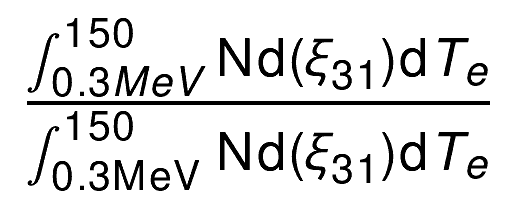# Graphics: TLatex latex string, missing denominator for frac and bad drawing alignment

Two issues to raise regarding TLatex

Here is a MWE

int latextest()
{

TCanvas *c = new TCanvas();

TLatex latex;
latex.SetNDC();
latex.SetTextFont(43);
latex.SetTextSize(20);

TString latexstring;
latexstring.Form("#frac{1}{2}");
latex.DrawLatex(0.1, 0.1, latexstring);

TString latexstring2;
latexstring2.Form("#frac{#int dX}{#int dX}");
latex.DrawLatex(0.1, 0.3, latexstring2);

TString latexstring3;
latexstring3.Form("#frac{#int^{5}_{0} dX}{#int^{5}_{0} dX}");
latex.DrawLatex(0.1, 0.5, latexstring3);

return 0;
}


Problems:

• latexstring2 draws the integrals in such a way that the denominator collides with the fraction line

• latexstring3 produces an error:

*ERROR<TLatex>: Missing denominator for #frac
==> #frac{#int^{5}_{0} dX}{#int^{5}_{0} dX}


I am not sure if the second issue is my own mistake or a problem with ROOT latex syntax interpreter.

First issue seems like a (probably) easy fix, or perhaps a workaround somehow? Screenshot below shows problem.

Hi,

maybe you could use TMathText instead of TLatex? It would fix both issues:

int latextest()
{

TCanvas *c = new TCanvas();

TMathText latex;
latex.SetNDC();
latex.SetTextFont(43);
latex.SetTextSize(20);

TString latexstring;
latexstring.Form("1 \\over 2");
latex.DrawMathText(0.1, 0.1, latexstring);

TString latexstring2;
latexstring2.Form("\\int dX \\over \\int dX}");
latex.DrawMathText(0.1, 0.3, latexstring2);

TString latexstring3;
latexstring3.Form("\\int^{5}_{0} dX \\over \\int^{5}_{0} dX");
latex.DrawMathText(0.1, 0.5, latexstring3);

return 0;
}



It could be a good solution. Is TMathText prefered to TLatex for some reason? What is the difference between the two?

So I have made some progress using your suggestion, however I encounter a further issue.

In the example below, the ^{150} replaces the superscript (upper) limit of the integral. This is supposed to preceed the isotope symbol Nd.

TString mtstring;
mtstring.Form("\\int_{0.3 MeV}^{5 MeV} ^{150}\\mathrm{Nd} (\\xi_{31}) \\mathrm{d}T_{e} \\over \\int_{0.3 \\mathrm{MeV}}^{5 \\mathrm{MeV}} ^{150}\\mathrm{Nd} (\\xi_{31}) \\mathrm{d}T_{e}");

mt.DrawMathText(0.1, 0.7, mtstring);


This is the result. Is there any way to prevent the symbol “150” from replacing that superscript?No idea. I’ll let more experienced people to answer that. But apparently sometimes TMathText does things TLatex can not.

I only came up with making 150 a right superscript of a space symbol coming right after the integral:

mtstring.Form("\\int_{0.3 MeV}^{5 MeV} \\xspace^{150}\\mathrm{Nd} (\\xi_{31}) \\mathrm{d}T_{e} \\over \\int_{0.3 \\mathrm{MeV}}^{5 \\mathrm{MeV}} \\xspace^{150}\\mathrm{Nd} (\\xi_{31}) \\mathrm{d}T_{e}");

It kinda works. Unfortunately, the way I’d usually do it with TLatex using {}^{150}Nd makes ROOT segfault for some reason when fed into TMathText.\\hbox{}^{150} doesn’t segfault for me and produces no space.
And you can use raw string literals if you have ROOT6 and need to type lotsa backslashes.

I found a solution: Enclose the first part (the “#int^…_…” bit) in braces {}

…and to fill in on TMathText vs TLatex: TMathText's rendering engine is superior. BUT it doesn’t support PDF< only PostScript. You’re welcome to submit a bug for each of the crashes and wrong parses you found at https://github.com/root-project/root/issues - thanks!

This topic was automatically closed 14 days after the last reply. New replies are no longer allowed.